IMO Shortlist 1994 problem N6

Kvaliteta:
Avg: 0,0
Težina:
Avg: 8,0
Define the sequence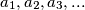$a_1, a_2, a_3, ...$ as follows.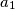$a_1$ and$a_2$ are coprime positive integers and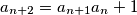$a_{n + 2} = a_{n + 1}a_n + 1$. Show that for every$m > 1$ there is an$n > m$ such that$a_m^m$ divides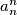$a_n^n$. Is it true that$a_1$ must divide$a_n^n$ for some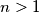$n > 1$?
Izvor: Međunarodna matematička olimpijada, shortlist 1994